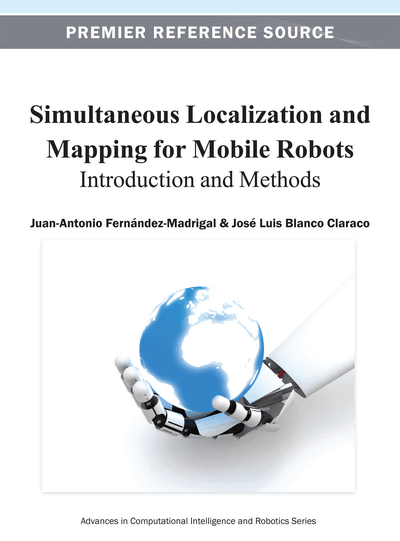# Probabilistic Bases

Copyright: © 2013 |Pages: 50
DOI: 10.4018/978-1-4666-2104-6.ch003
OnDemand PDF Download:
Available
\$37.50
No Current Special Offers

## Abstract

This is the third chapter of the first section. It is a compendium of all the concepts and theorems of probability theory that are found in the problems of Bayesian estimation of a robot location and the map of its environment. It presents uncertainty as an intrinsic feature of any mobile robot that develops in a real environment. It is then discussed how uncertainty has been treated along the history of science and how probabilistic approaches have represented such a huge success in many engineering fields, including robotics. The fundamental concepts of probability theory are discussed along with some advanced topics needed in further chapters, following a learning curve as smooth and comprehensive as possible.
Chapter Preview
Top

## Chapter Guideline

• You will learn:

• o

Why dealing explicitly with uncertainty is crucial for the problems of localization and mapping.

• o

A brief overview of the history of probability and statistics.

• o

The foundations of representing uncertainty with probability theory: probability spaces, random variables, probability distributions, pdfs, and pmfs.

• o

How uncertainty can be summarized: moments, information, and entropy.

• o

How to deal with multiple random variables simultaneously.

• o

The special role of the chi-squared distribution.

• o

On the dependence between random variables and the concept of conditional probability.

• o

How to use graphical models to represent dependences.

• Provided tools:

• o

A table with the most common probability density functions in mobile robotics and their parameters.

• o

Method of marginalization.

• o

Methods for propagating uncertainty through arbitrary functions and some particular cases of such transformations.

• o

Basic tools of conditional probability: Bayes' rule, chain rule, and law of total probability.

• o

The graphical criterion of d-separation to factor a distribution over a large numbers of random variables.

• o

The graph of correlations as a purely graphical method to reveal the sparseness of the relationships existing between the unknown random variables in any inference problem.

• Relation to other chapters:

• o

This chapter provides the basic foundations of probability required for the rest of the book.

• o

Transformation of r.v.s through a function (section 8) and graphical models (section 10) are pervasively employed in subsequent chapters.

Top

## 1. Introduction

After studying the nuts and bolts of mobile robots in chapter 2, we now move to a different perspective: the theoretical foundations of probabilistic mobile robot localization and mapping required for a proper and solid understanding of the methods that address these problems.

As discussed in chapter 1, one of the core issues that were preventing robotics engineers from proposing a consistent theoretical solution to practical localization and mapping before the nineties was how to deal with uncertainty. Uncertainty takes the form of noise in the data being processed, and comes from different sources exhibiting diverse behaviors. Ultimately, this reflects our lack of knowledge about the processes involved in perception and actuation of mobile robots (although quantic physics teaches us that at extremely small scales, uncertainty becomes an intrinsic feature of reality). Since we cannot build perfect models for those processes of our interest, it becomes mandatory to deal with their randomness in order to discern significant data from noise or errors.

## Complete Chapter List

Search this Book:
Reset